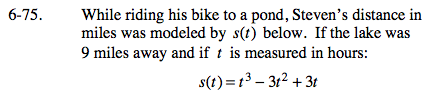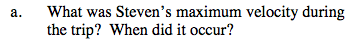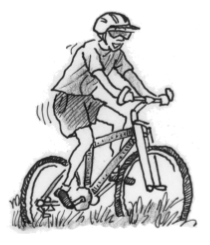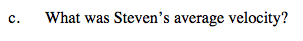### Home > CALC > Chapter 6 > Lesson 6.2.2 > Problem6-75

6-75.
1. While riding his bike to a pond, Steven's distance in miles was modeled by s(t) below. If the lake was 9 miles away and if t is measured in hours: Homework Help ✎
s(t) = t3 − 3t2 + 3t

1. What was Steven's maximum velocity during the trip? When did it occur?

2. Did Steven ever stop during the trip? Justify your conclusion analytically.

3. What was Steven's average velocity?v(t) = 3t2 − 6t + 3 = 3(t − 1)2

Visualize v(t). It is a concave up parabola; therefore there cannot be a local maximum. But Steven didn't ride his bicycle forever! You can still find the maximum velocity during the time interval when Steven was on his bike... Check the Endpoints!Does v(t) ever equal 0 during the time interval of Steven's bike ride?

$\text{Average velocity }=\frac{\text{displacement}}{\text{time}}$